• 光在吸收性介质界面反射系数和透射系数的研究，张秋长，罗天舒，通过定义吸收性介质中光波矢量的等幅面单位矢量和等相面单位矢量．分别应用相应的边界条件，获得了光在介质/吸收性介质、吸收性�
• 在震相分析中，应力的反射系数和透射系数对于震相初动的判断有着重要的作用。本文主要给出了应力的反射系数和透射系数的计算公式。
• 计算多层媒质的反射和透射系数，输入是多层媒质的层数、每层的厚度、每层的介电常数磁导率，输出是最外层的反射系数
• 采用以阶梯形势垒代替施瓦希-德希特时空中视界附近的势垒的方法,计算了此时空中标量场辐射的反射系数和透射系数。结果表明在此时空中,标量场的反射系数趋于零,而透射系数接近于1,即所有的标量场都将透过势垒而传播到...
• 入射光的电矢量总是可以分解为垂直于入射面的s波与平行于入射面的p波，求得s波与p波的反射系数透射系数后，可以用matlab拟合出系数曲线。 %figure 1.23 calculation of rs rp ts tp u_1=0:0.5:90; u_2=asind...
入射光的电矢量总是可以分解为垂直于入射面的s波与平行于入射面的p波，求得s波与p波的反射系数及透射系数后，可以用matlab拟合出系数曲线。
%figure 1.23 calculation of rs rp ts tp
u_1=0:0.5:90;
u_2=asind(sind(u_1)/1.5);
r_s=sind(u_2-u_1)./sind(u_2+u_1);
t_s=2*sind(u_2).*cosd(u_1)./sind(u_1+u_2);
r_p=tand(u_1-u_2)./tand(u_1+u_2);
t_p=2*sind(u_2).*cosd(u_1)./(sind(u_1+u_2).*cosd(u_1-u_2))
plot(u_1,r_s)
hold on
plot(u_1,t_s)
hold on
plot(u_1,r_p)
hold on
plot(u_1,t_p)
title('s波与p波的反射系数与透射系数曲线')
grid
xlabel('角度')
ylabel('比值')
text(28,-0.23474,'rs')
text(31,0.15575,'rp')
text(57,0.60912,'ts')
text(73.5,0.47532,'tp')
%legend('rs','rp','ts','tp')

展开全文matlab
• 给定入射角、偏振、波长、每层的复折射率每层的厚度，该程序生成多层叠层的复反射和透射系数。 该程序假定介质入射出射介质是无损的，但薄膜层可能是有损的（这是由折射率的复杂性质造成的）。 该程序还假定所有...matlab
• 基于MATLAB软件，使用者在交互界面GUI上输入入射介质、反射介质的折射系数n1、n2，可算得不同入射角条件下线偏光/自然光的菲涅尔透反射系数及透反射比
• 研究了两种介质分界面存在面电荷分布时的反射与透射，证明了这一条件下反射定律和折射定律仍然成立，推导得到存在面电荷分布时入射波平行分量和垂直分量的振幅反射系数和振幅透射系数，即修正的菲涅耳公式。...
• 不同角度入射的反射和传递系数估计取决于材料及其宽度。 Aslo取决于极化。matlab
• 编写程序计算入射P波S波在固-固界面上垂直入射的位移反射和透射系数，能量比值。利用程序估算核-幔边界（尽管是固-液界面）处的这些物理量，假定下地幔p波速度为13.7km/s，S波速度为7.2km/s，密度为5.5g/cm³，地...
• C-1 对于给定的模型，追踪不同的入射角的射线路径，计算射线参数，走时，距离，截距。画出射线路径，走时-距离曲线，射线参数-距离曲线，Tau-P曲线。
• 声波透过多层介质的反射系数，计算声波透过多层介质的反射系数和透射系数
• 网络输入端反射系数 Γin\Gamma_{in}Γin​ Γin=b1a1=S11+S12a2a1\Gamma_{in}=\frac{b_1}{a_1}=S_{11}+S_{12}\frac{a_2}{a_1}Γin​=a1​b1​​=S11​+S12​a1​a2​​ 1/ΓL=b2a2=S21a1a2+S221/\Gamma_{L}=\frac...
网络输入端反射系数

Γ

i

n

\Gamma_{in}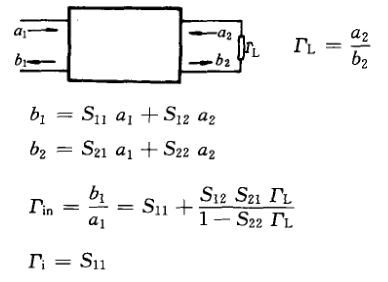Γ

i

n

=

b

1

a

1

=

S

11

+

S

12

a

2

a

1

\Gamma_{in}=\frac{b_1}{a_1}=S_{11}+S_{12}\frac{a_2}{a_1}

1

/

Γ

L

=

b

2

a

2

=

S

21

a

1

a

2

+

S

22

1/\Gamma_{L}=\frac{b_2}{a_2}=S_{21}\frac{a_1}{a_2}+S_{22}

1

/

Γ

L

−

S

22

S

21

=

a

1

a

2

\frac{1/\Gamma_{L}-S_{22}}{S_{21}}=\frac{a_1}{a_2}

Γ

i

n

=

S

11

+

S

12

a

2

a

1

=

S

11

+

S

12

S

21

1

/

Γ

L

−

S

22

\Gamma_{in}=S_{11}+S_{12}\frac{a_2}{a_1}=S_{11}+S_{12}\frac{S_{21}}{1/\Gamma_{L}-S_{22}}

Γ

L

=

0

\Gamma_{L}=0

得插入反射系数

Γ

i

=

S

11

\Gamma_{i}=S_{11}


展开全文•研究论文
• 研究了光从各向同性介质入射至单轴晶体界面上时反射折射情况，采用一套较为简单的公式计算方法，再加上坐标轴的旋转，从而给出了在晶体光轴取向任意的一般情况下，光在晶体界面上的透射反射系数的具体表达式。
• 传输矩阵法，一般用来研究周期性分层介质中的传输特性。对于分层媒质的微分方程在特定条件下的解，最简单便捷的方式便是使用传输矩阵。传输矩阵法就是用矩阵的形式来描述电磁波在多层介质中的传播情况，在每一层介质...matlab
• 并对层状模型逐步简化,依次给出了薄层、单阻抗差界面纵波入射时的反射透射系数公式,指出了位移与位移位函数这两种不同形式下各公式的异同适用条件,对于薄层地震反演精度的提高具有一定的指导作用。
• 计算多层媒质的反射和透射系数,多层介质膜的反射率,matlab源码
• 梳理吸收率、反射和透射率吸收率 α\alphaα反射率 $\rho$透射率 τ\tauτ 【缘起】一个物体从太阳接收辐射能量，这部分辐射能到达物体之后的归宿是什么样子的呢？不同的物体对于辐射的表现又有什么差异呢？这...


梳理吸收率、反射率和透射率
吸收率

α

\alpha

反射率

ρ

\rho

透射率

τ

\tau

相关性质物体净辐射吸收

【缘起】一个物体从太阳接收辐射能量，这部分辐射能到达物体之后的归宿是什么样子的呢？不同的物体对于辐射的表现又有什么差异呢？这需要用吸收率、反射率和透射率来说明。

首先，三者的关系为

α

+

ρ

+

τ

=

1

\alpha + \rho + \tau = 1

吸收率

α

\alpha

absorptivity, 指的是入射辐射能被物体所吸收的部分所在的比率，无量纲。
反射率

ρ

\rho

reflectivity, 指的是入射辐射能被物体所反射（包括镜面反射和漫反射）的部分所在的比率，无量纲。
透射率

τ

\tau

transmissivity, 指的是入射辐射能穿透过物体所的部分所在的比率，无量纲。

相关性质
上述3种指标是和波长有关的光学属性。例如，绿色植被的叶片，他对于太阳辐射中的可见光部分的吸收率可以达到85%，但是对于近红外波段的吸收率却不足50%；对于充分厚且不透明的物体，其透射率

τ

\tau

= 0，此时有

α

+

ρ

=

1

\alpha + \rho = 1

；根据 Kirchhoff’s 定律，对于特定波长的光，物体对其的 吸收率

α

\alpha

= 发射率

ϵ

\epsilon

。因此，对于黑体（Blackbody）而言，其

α

\alpha

=

ϵ

\epsilon

= 1， 其

ρ

=

τ

=

0

\rho = \tau = 0

.一个不透明的灰体，

τ

=

0

\tau=0

，此时有

α

+

ρ

=

1

\alpha + \rho = 1

；

物体净辐射吸收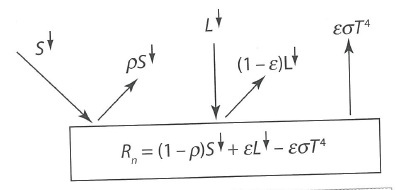上图为一个不透明灰体（实际中最常见到的情况）的辐射平衡示意图。图中，物体收到来自太阳的短波辐射（Solar）和来自大气的长波辐射（Longware）。

S

↓

S↓

表示短波辐射

ρ

S

↓

\rho S↓

表示物体反射的短波辐射

L

L

表示长波辐射

(

1

−

ϵ

)

L

(1-\epsilon)L

表示辐射的长波辐射，

ϵ

L

\epsilon L

为物体对长波辐射的吸收量
只要温度大于绝对零度的物体本身也会向外界发射长波辐射，其辐射能力与自身的温度有关系，因此，物体的净辐射吸收为

R

n

+

(

1

−

ρ

)

S

↓

+

ϵ

L

↓

−

ϵ

σ

T

4

R_n + (1-\rho)S↓ + \epsilon L↓ - \epsilon\sigma T^4

其中，

ρ

\rho

为对所有波段的反射能力，通常也被称为地表反照率（surface albedo）,

ϵ

\epsilon

为发射率。

σ

\sigma

为stefan-Boltzman常数，常取值为

σ

=

5.67

×

1

0

−

8

W

m

−

2

K

−

4

\sigma = 5.67×10^{-8}Wm^{-2}K^{-4}展开全文• 导出了平面波在双折射介质表面的反射系数和透射系数公式。并发展了一种计算多层膜系统(其中若干膜层或基底是双折射材料)反射率随表面极坐标分布的公式和数值方法。
• 主要研究了在三维物性界面上的平面波入射的反射与透射系数，用mathematic数学软件获得反射系数透射系数较复杂的计算公式，并做了数值计算，对工程应用可作参考。
• 利用Blot多孔介质声传播理论对声波在水多孔海底界面上的反射透射进行了分析，具体给出反射声波的反射系数，3种透射声波的透射系数以及声能透射系数随入射波入射角频率（10一40kHz）的变化关系。分析了各种透射波...
• 通过理论计算获得了全反射时分层介质中p偏振光的透射系数计算公式, 并采用时域有限差分(FDTD)方法进行了验证。计算所获得的公式与传统的采用分层介质的特性矩阵法计算获得的相似, 不同之处在于当发生全反射时, 公式...
• 透/反射率的计算在电磁波研究中非常常见，计算结果的准确性与材料参数定义，边界条件的选择，网格剖分有十分紧密的关系。以下是个人关于电磁波透/反射率计算问题的经验整理，如有错漏欢迎指正补充。
• 该程序可用来求解多层倾斜界面中的波传播的反透射系数
• MATLAB高等光学仿真——反射率，透射率变化 1.subplot（m,n,p）或者subplot（m n p）。 subplot是将多个图画到一个平面上的工具。其中，m表示是图排成m行，n表示图排成n列，也就是整个figure中有n个图是排成一行的，...
MATLAB高等光学仿真——反射率，透射率变化
1.subplot（m,n,p）或者subplot（m n p）。 subplot是将多个图画到一个平面上的工具。其中，m表示是图排成m行，n表示图排成n列，也就是整个figure中有n个图是排成一行的，一共m行，如果m=2就是表示2行图。p表示图所在的位置，p=1表示从左到右从上到下的第一个位置。subplot(m,n,p) 是画一张图中包含若干子图，每个子图也是连续的，可将多个图画到一个平面上。 用法如下所示：由以上我们可以看到，他是可以把好几张图画到同一figure里面。 2.stem() 函数用来画一张整图，画出来是离散函数。plot() 是连续函数，可以画一张连续的图；最一般最常用的画法。 3.abs()函数的三大功能
求实数的绝对值求复数的模求字符串的ASCII码值。
4.legend()函数是图例注释函数，一般用法为legend(线性1，线性2，…参数字符串…)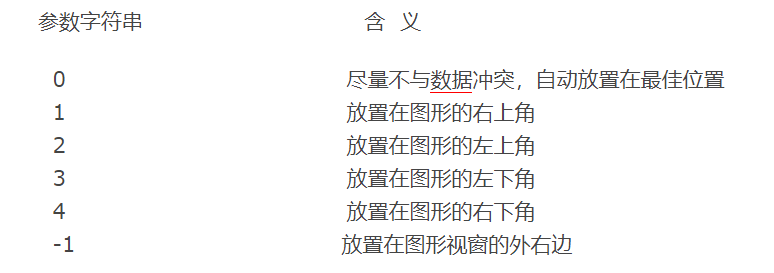5.num2str()函数的使用方法
str = num2str(A)即将数字A转换成char类型，值得注意的是A也可以是数字类型的数组。str = num2str(A, precision) A: 数值类型的数组或者是单个的数值 precision：转换成字符穿保留的数字位数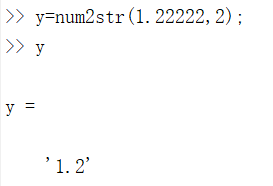str = num2str(A, format) A: 数值类型的数组或者是单个的数值 format:指定数字转换为字符串的格式，通常’%11.4g’是默认的。 也可以指定转换为几位的字符串，不足用0填充，如%02d， %03d等。 6.axis( [xmin xmax ymin ymax] )函数：可以设置当前坐标轴 x轴 和 y轴的限制范围。 7.grid on表示作图加栅格。
程序如下：
clear%清空内存空间 clear all%关闭所有作图页面
n1=1,n2=1.45; theta=0:0.1:90;%入射角范围，步距0.1° a=theta*pi/180;%角度化为弧度
rp=(n2cos(a)-n1sqrt(1-(n1/n2sin(a)).^2))./… (n2cos(a)+n1sqrt(1-(n1/n2sin(a)).^2)); rs=(n1cos(a)-n2sqrt(1-(n1/n2sin(a)).^2))./… (n1cos(a)+n2sqrt(1-(n1/n2sin(a)).^2)); tp=2n1cos(a)./(n2cos(a)+n1sqrt(1-(n1/n2sin(a)).^2)); ts=2n1cos(a)./(n1cos(a)+n2sqrt(1-(n1/n2sin(a)).^2));
figure(1); subplot(1,2,1);%画第一行第一列第一个图 plot(theta,rp,’-’,theta,rs,’–’,theta,abs(rp),’:’,… theta,abs(rs),’-.’,‘LineWidth’,2); legend(‘r_p’,‘r_s’,‘t_p’,‘t_s’); xlabel(’\theta_i’); ylabel(‘AM’); title([‘n_1=’,num2str(n1),‘n_2=’,num2str(n2)]); axis([0,90,-1,1]); grid on
subplot(1,2,2);%画第一行第一列第一个图 plot(theta,tp,’-’,theta,ts,’–’,theta,abs(tp),’:’,… theta,abs(ts),’-.’,‘LineWidth’,2); legend(‘r_p’,‘r_s’,‘t_p’,‘t_s’); xlabel(’\theta_i’); ylabel(‘AM’); title([‘n_1=’,num2str(n1),‘n_2=’,num2str(n2)]); axis([0,90,0,1]); grid on
结果显示如下：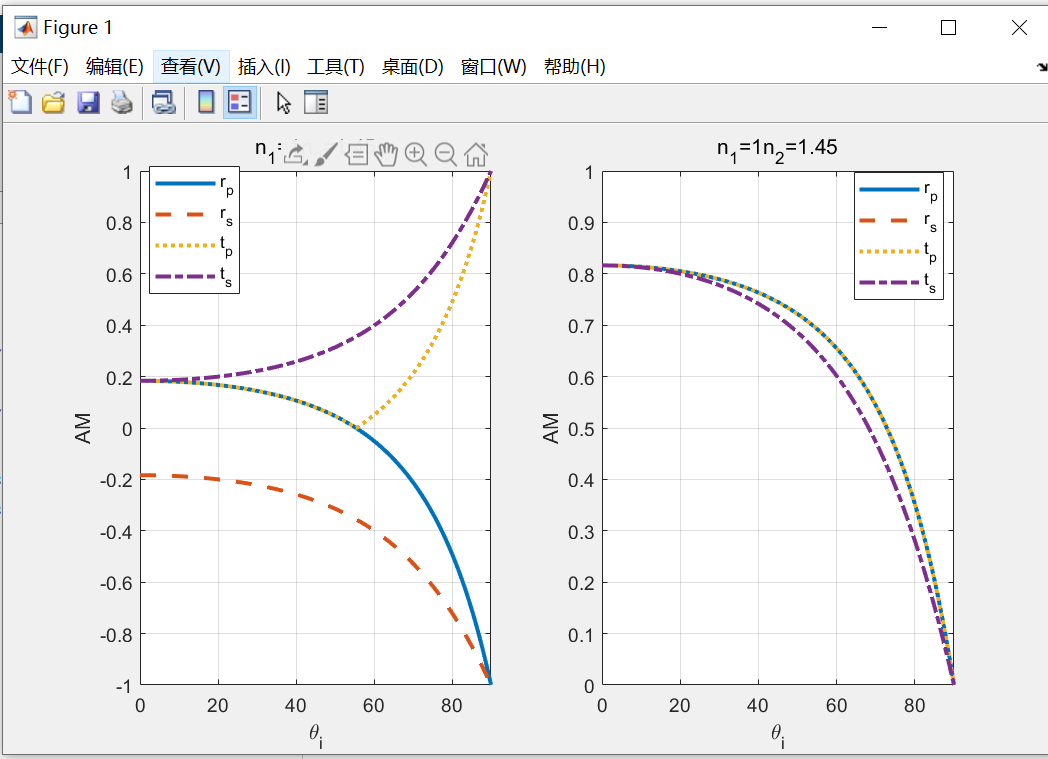展开全文matlab
• 网上关于FEKO仿真多层介质反射系数的资料很少，经过多方探索以及采坑，总结了一下，记录在此以方便以后查看。 ——二〇一七年三月二十六日 此处仿真为放置于空气中的两层介质板的反射系数，多层同理。介质参数的......